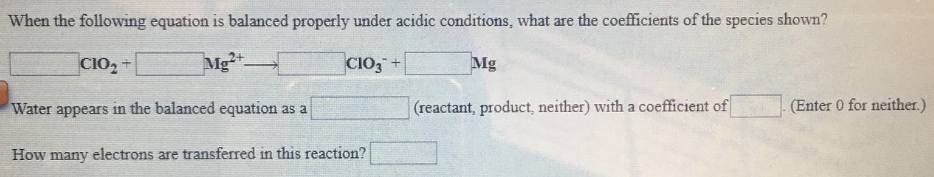# When the following equation is balanced properly under acidic conditions, what are the coefficients of the species shown? ___ ClO^2 + ___ Mg^2+ → ___ ClO3^- + ___ Mg Water appears in the balanced equation as a ____ (reactant, product, neither) with a coefficient of ____. (Enter 0 for neither.) How many electrons are transferred in this reaction?